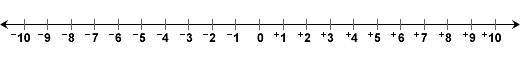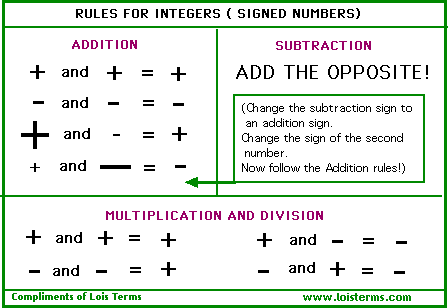# rule of integersProblem: George owes his friend Jeanne \$3. If he borrows another \$6, how much will he owe her altogether?

Solution: This problem is quite simple: just add \$3 and \$6 and the result is \$9.

The problem above can be solved using addition of integers. Owing \$3 can be represented by -3 and owing \$6 can be represented by -6. The problem becomes:
-3 + -6 = -9

Look at the number line below. If we start at 0, and move 3 to the left, we land on -3. If we then move another 6 to the left, we end up at -9.Rule: The sum of two negative integers is a negative integer.

Example 1: Find the sum of each pair of integers. You may draw a number line to help you solve this problem.

 Adding Negative Integers Integers Sum -2 + -9 = -11 -5 + -8 = -13 -13 + -7 = -20

Do not confuse the sign of the integer with the operation being performed. Remember that:-2 + -9 = -11 is read as Negative 2 plus negative 9 equals negative 11.

Rule: The sum of two positive integers is a positive integer.

Example 2: Find the sum of each pair of integers. You may draw a number line to help you solve this problem.

 Adding Positive Integers Integers Sum +2 + +9 = +11 +17 + +5 = +22 +29 + +16 = +45

Do not confuse the sign of the integer with the operation being performed. Remember that:

+29 + +16 = +45 is read as Positive 29 plus positive 16 equals positive 45.

So far we have added integers with like signs (either both negative or both positive). What happens when we add integers with unlike signs? How do we add a positive and a negative integer, or a negative and a positive integer?

Procedure: To add a positive and a negative integer (or a negative and a positive integer), follow these steps:

1. Find the absolute value of each integer.

2. Subtract the smaller number from the larger number you get in Step 1.

3. The result from Step 2 takes the sign of the integer with the greater absolute value.

We will use the above procedure to add integers with unlike signs in Examples 3 through 7. Refer to the number line to help you visualize the process in each example. We will use money as an alternative method for adding integers.

Example 3: Find the sum of +7 and -4.Step 1: |+7| = 7 and |-4| = 4

Step 2: 7 - 4 = 3

Step 3: The number 3 will take a positive sign since +7 is farther from zero than -4.

Solution 1: +7 + -4 = +3

Solution 2: If you start with \$7 and you owe \$4, then you end up with \$3.

Example 4: Find the sum of -9 and +5.Step 1: |-9| = 9 and |+5| = 5

Step 2: 9 - 5 = 4

Step 3: The number 4 will take a negative sign since -9 is farther from 0 than +5.

Solution 1: -9 + +5 = -4

Solution 2: If you owe \$9 and you are paid \$5, then you are still short \$4.

Example 5: Find the sum of +6 and -7.Step 1: |+6| = 6 and |-7| = 7

Step 2: 7 - 6 = 1

Step 3: The number 1 will take a negative sign since -7 is farther from 0 than +6.

Solution 1: +6 + -7 = -1

Solution 2: If you start with \$6 and you owe \$7, then you are still short \$1.

Example 6: Find the sum of -6 and +7.Step 1: |-6| = 6 and |+7| = 7

Step 2: 7 - 6 = 1

Step 3: The number 1 will take a positive sign since +7 is farther from 0 than -6.

Solution 1: -6 + +7 = +1

Solution 2: If you owe \$6 and you are paid \$7, then you end up with \$1.

Example 7: Find the sum of +9 and -9.Step 1: |+9| = 9 and |-9| = 9

Step 2: 9 - 9 = 0

Step 3: The integer 0 has no sign.

Solution 1: +9 + -9 = 0

Solution 2: If you start with \$9 and you owe \$9, then you end up with \$0.

In Example 7 you will notice that the integers +9 and -9 are opposites. Look at the problems below. Do you see a pattern?

-100 + +100 = 0

+349 + -349 = 0

-798 + +798 = 0

Rule:   The sum of any integer and its opposite is equal to zero.

Summary: Adding two positive integers always yields a positive sum; adding two negative integers always yields a negative sum. To find the sum of a positive and a negative integer, take the absolute value of each integer and then subtract these values. The result takes the sign of the integer with the larger absolute value. The sum of any integer and its opposite is equal to zero.### Exercises

Directions: Read each question below. Click once in an ANSWER BOX and type in your answer; then click ENTER. After you click ENTER, a message will appear in the RESULTS BOX to indicate whether your answer is correct or incorrect. To start over, click CLEAR.

 1.-6 + -9 = ? ANSWER BOX:    RESULTS BOX:
 2.+7 + +11 = ? ANSWER BOX:    RESULTS BOX:
 3.+5 + -3 = ? ANSWER BOX:    RESULTS BOX:
 4.-2 + +7 = ? ANSWER BOX:    RESULTS BOX:
 5.+9 + -12 = ? ANSWER BOX:    RESULTS BOX: